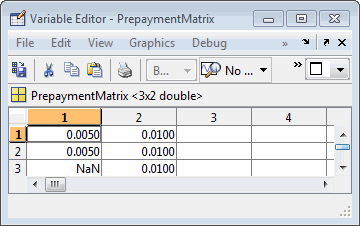## Pools with Different Numbers of Coupons Remaining

Suppose that one pool has two remaining coupons, and the other has three. MATLAB® expects the prepayment matrix to be in the following format:

```V11 V21 V12 V22 NaN V23 ```

`V``ij` denotes the single monthly mortality (SMM) rate for pool `i` during the `j`th coupon period since `Settle`.

The use of `NaN` to pad the prepayment matrix is necessary because MATLAB cannot concatenate vectors of different lengths into a matrix. Also, it can serve as an error check against any unintended operation (any MATLAB operation that would return `NaN`).

For example, assume that the 2-month pool has a constant SMM of 0.5% and the 3-month pool has a constant SMM of 1% in every period. The prepayment matrix you would create is depicted below.Create this input in whatever manner is best for you.

### Summary of Prepayment Data Vector Representation

• When you specify a PSA prepayment speed, MATLAB "seasons" the pool according to its age.

• When you specify your own prepayment matrix, identify the maximum number of coupons remaining using `cpncount`. Then supply the matrix elements up to the point when cash flow ceases to exist.

• When different length pools must exist in the same matrix, pad the shorter one(s) with `NaN`. Each column of the prepayment matrix corresponds to a specific pool.